Definitions of Square Dance Calls and Concepts

Index -->  Plus  |  A1  |  A2  |  C1  |  C2  |  C3A  |  C3B  |  C4  |  NOL  |
Definitions (Text Only) -->  Plus  |  A1  |  A2  |  C1  |  C2  |  C3A  |  C3B  |  C4  |  NOL  |
 Find call:

Tandem \$B\$N(B 2 \$B?M(B, \$B8~\$+\$\$9g\$C\$?(B 2 \$B?M(B, \$B\$"\$k\$\$\$OGXCf9g\$o\$;\$N(B 2 \$B?M\$+\$i!%(B

Right Roll To A Wave \$B\$r\$7\$F(B, Arm Turn 1/4 (\$B\$"\$k\$\$\$O;X<(\$5\$l\$?(B fraction) \$B\$r\$7\$^\$9!%(B

\$B1&\$N(B Mini-Wave \$B\$G=*\$o\$j(B, 2 \$B%Q!<%H\$N%3!<%k\$G\$9!%(B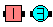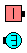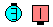Latch On\$B\$NA0(B Right Roll To A Wave\$B\$N8e(B Arm Turn 1/4\$B\$N8e(B (\$B=*\$o\$j(B)

\$B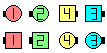\$B\$B;O\$a\$N(B formation: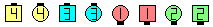As Couples Once Removed Latch On\$B\$N8e(B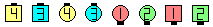Once Removed As Couples Latch On\$B\$N8e(B

1. \$B;O\$a\$N>l9g(B, 'As Couples' \$B\$,:G=i\$KJ9\$3\$(\$k\$N\$G(B, As Couples \$B\$G(B 'Once Removed Latch On' \$B\$N%3!<%k\$r\$7\$^\$9!%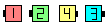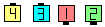1 \$B?M(B 1 \$B?M\$,(B couple \$B\$@\$H;W\$C\$F(B\$B%3!<%k\$r;O\$a\$^\$9(B Once Removed Latch On\$B\$N8e(B
2. 2 \$B\$DL\\$N>l9g(B, 'Once Removed' \$B\$,:G=i\$KJ9\$3\$(\$k\$N\$G(B, Once Removed \$B\$G(B 'As Couples Latch On' \$B\$N%3!<%k\$r\$7\$J\$1\$l\$P\$J\$j\$^\$;\$s!%\$3\$N>l9g\$KCm0U\$9\$k\$3\$H\$O(B, {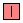,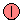,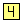,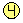} \$B\$N?M\$,(B, \$B;O\$a\$H=*\$o\$j\$NN>J}\$N(B formation \$B\$GF1\$8Cg4V\$G(B, Once Removed \$B\$N0LCV\$K\$\$\$J\$1\$l\$P\$J\$j\$^\$;\$s!%F1MM\$K(B, {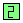,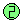,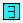,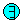} \$B\$N?M\$,F1\$8Cg4V\$G\$9!%(BCALLERLAB definition for Latch On (fraction)Choreography for Latch On (fraction)Comments? Questions? Suggestions?

https://www.ceder.net/def/latchon.php?language=japan
19-September-2020 11:35:22